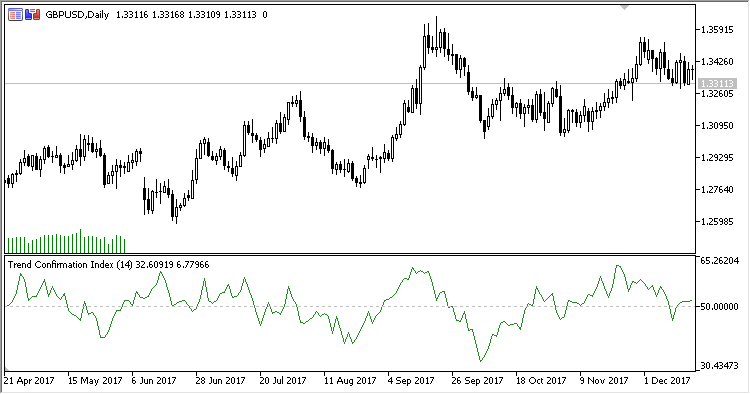Interesting script?
So post a link to it -
let others appraise it

You liked the script? Try it in the MetaTrader 5 terminal# TCI - indicator for MetaTrader 5

Views:
1506
Rating:
Published:
2018.07.09 11:05
Updated:
2018.09.17 10:57

Indicator "Trend Confirmation Index"

If we use an assumption that theclose price location within a candlestick contains information of whether the trend is strong or weak and whether it gains or loses its power, then we can calculate the current market state - a trend confirmation index (TCI).

Indicator TCI calculates the average value of the close price location within the last N candlesticks.
Close price location within a candlestick is calculated as (C-L) / ((H-L) / 100)

The indicator has five configurable parameters:

• Period - calculation period
• Method - calculation method
• Overbought level - overbought level
• Oversold level - oversold level
• Show actual CPP - whether to display the close price location line (Closing price position) for each candlestick or not (Yes/No)

Calculation:

```TCI = MA(CPP)
```

where:

```CPP = 100.0 * (Close-Low) / (High-Low)
MA is the moving average with the Method calculation type and the Period calculation period
```Translated from Russian by MetaQuotes Software Corp.
Original code: https://www.mql5.com/ru/code/20992Speed_of_tradeReverse_MACDVolatilityMAWAMI